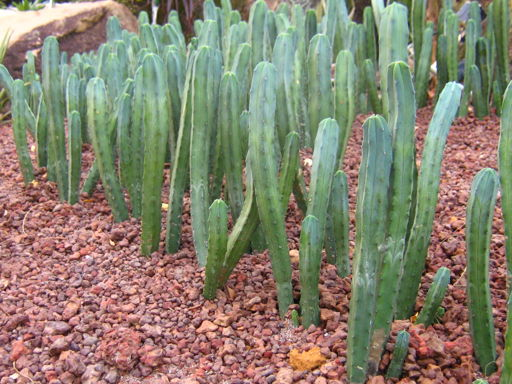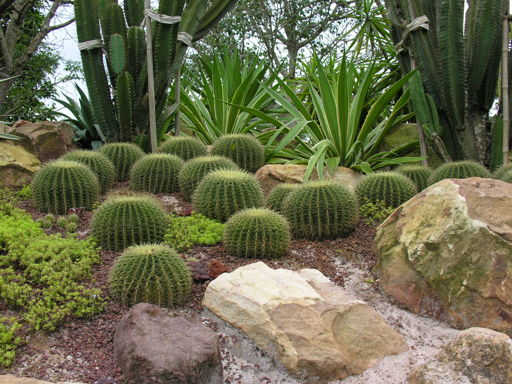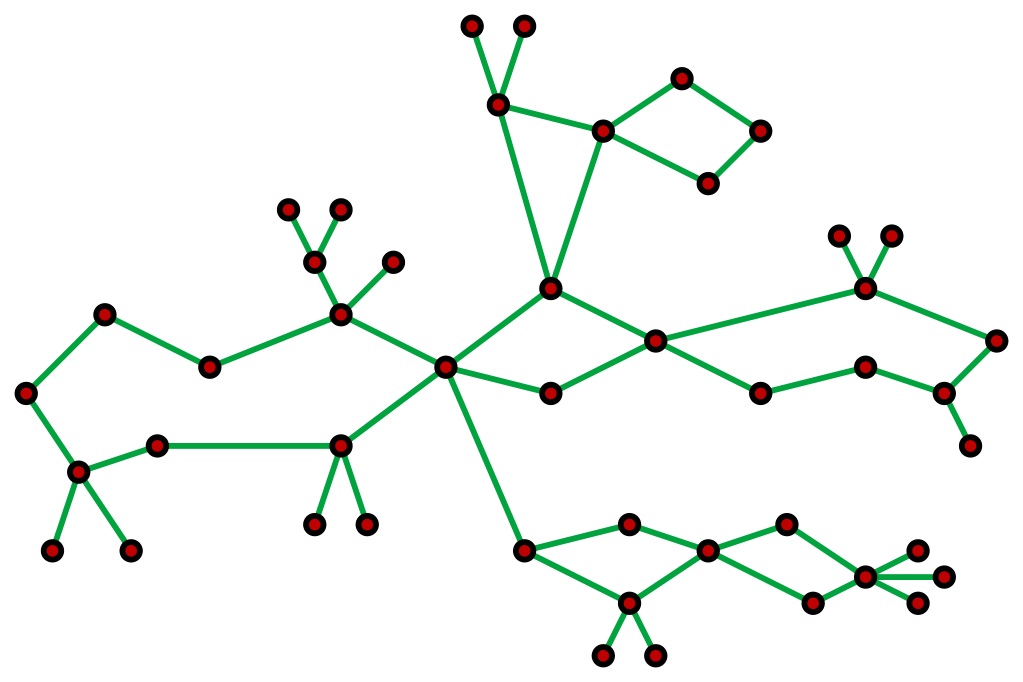시간 제한 메모리 제한 제출 정답 맞은 사람 정답 비율
5 초 1024 MB 1 1 1 100.000%

## 문제

After retirement, UT CS professors go to a farm in upstate Texas where they garden and do research to their hearts' content. Oftentimes these two interests overlap, as is the case with the amazing Upstate Texas Cactus Shoppe (UTCS).

For those of us who are not retired CS professors, a cactus is an undirected graph where each edge belongs to at most one simple cycle. Here are some pictures of cactuses:Figure 1: Cactuses! Left photo by Calvin Teo. Right photo by Genleorus.

Here is a picture of a cactus graph:Figure 2: Cactus graph! Image created by David Eppstein.

The UTCS has a single cactus. Each vertex in the cactus has a flower identifier $f_i$, describing the flowers that reside in that part of the cactus. If $f_i$ and $f_j$ share divisors, then the flowers at those vertices are similar.

To conduct a purchase at the UTCS, a professor comes in with a flower identifier $q_i$. They then make a copy of the master cactus and remove all the vertices where $q_i$ does not divide $f_i$. They also remove all edges which were attached to at least one deleted vertex. Afterwards, the cactus may become disconnected. Each professor wonders how many connected components they are left with after this process. An empty graph has $0$ connected components. Two vertices are in the same connected component if there is some simple path using the remaining edges from one vertex to the other.

All this cutting and pruning is hard work, especially for the retired. Can you help answer their queries?

## 입력

The first line has three space-separated integers $n$ ($2 \leq n \leq 100\,000$), $m$ ($1 \leq m \leq 200\,000$) and $q$ ($1 \leq q \leq 100\,000$): the number of vertices in the cactus, the number of edges in the cactus and the number of queries. The next line has $n$ space separated integers $f_i$ ($1 \leq f_i \leq 1\,000\,000$): the flower identifier for each vertex. The next $m$ lines each contain two integers $a$ and $b$ ($1 \leq a, b \leq n$), meaning there is an edge connecting $a$ and $b$. It is guaranteed these edges form a connected valid cactus. No edge appears more than once and no edge connects a vertex with itself.

The next $q$ lines each contain a professor's query $q_i$ ($1 \leq q_i \leq 1\,000\,000$).

## 출력

For each query made by a professor, output the number of connected components that remain when removing all the vertices that are not divisible by $q_i$.

## 예제 입력 1

3 2 5
15 10 6
1 2
2 3
5
14
10
3
2


## 예제 출력 1

1
0
1
2
1


## 예제 입력 2

5 6 4
6 15 9 20 12
1 2
2 3
3 1
2 4
4 5
5 2
5
3
7
2


## 예제 출력 2

1
1
0
2


## 출처• 문제를 만든 사람: Arnav Sastry, Ethan Arnold